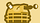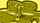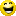cancel
Showing results for
Did you mean:

## x_currency_code & x_fp_hash

Does anyone know How to generate a correct x_fp_hash that includes the x_currency_code field ?

Right now I am doing it like this:

OpenSSL::HMAC.hexdigest( OpenSSL::Digest::Digest.new('md5'), @gateway.transaction_key, "#{@gateway.login.to_s.rstrip}^#{rand((1 << 32 ) - 1).to_s.rstrip}^#{x_fp_sequence.to_s.rstrip}^#{@order.total.to_s.rstrip}^#{@order.currency.to_s.rstrip}^" )

and I get an 99 error code...Contributor
2 ACCEPTED SOLUTIONS

Accepted Solutions
`id="x_ammount" `

should be x_amountOh ... lol ... if this is the mistake I'll kick myself.

LE: Basically there were two problems :

1. The silly typo for amount

2. The fact that authorize.net requires two decimals format for amountContributor
9 REPLIES 9

http://developer.authorize.net/guides/SIM/wwhelp/wwhimpl/js/html/wwhelp.htm#href=SIM_Submitting_tran...

Please note the required trailing “^” character. If you specify x_currency_code, then the value (for example, LVL) must be placed after the trailing caret.
 Example Fingerprint input with currency code specified
"authnettest^789^67897654^10.50^LVL"

you have the ending caret which shouldn't be there.Expert

I tried removing the trailing ^ now ,  but it doesn't seem to be working... but thanks for the like, it's spot on, I am probably doing something wrong.

Do you have any idea if the following is the right order for the parameters ?

OpenSSL::HMAC.hexdigest( OpenSSL::Digest::Digest.new('md5'), @gateway.transaction_key, "#{@gateway.login.to_s.rstrip}^#{x_fp_sequence.to_s.rstrip}^#{x_fp_timestamp.to_s.rstrip}^#{@order.total.to_s.rstrip}^#{@order.currency.to_s.rstrip}" ) %>ContributorI have tried and I always get different results than the ones from the browser.Contributor

Do you have any idea if the following is the right order for the parameters ?

OpenSSL::HMAC.hexdigest( OpenSSL:igest:igest.new('md5'), @gateway.transaction_key, "#{@gateway.login.to_s.rstrip}^#{x_fp_sequence.to_s.rstrip}^#{x_fp_timestamp.to_s.rstrip}^#{@order.total.to_s.rstrip}^#{@order.currency.to_s.rstrip}" ) %>

the order look right. I'm not familiar with the Ruby coding. could you debug to see if it got data correctly?Well ... cannot really since x_fp_hash is not returned as a param

`<input class="textInput" id="x_login" name="x_login" type="hidden" value="4s8QPsNt9a" /><input class="textInput" id="x_ammount" name="x_ammount" type="hidden" value="111.0" /><input class="textInput" id="x_fp_sequence" name="x_fp_sequence" type="hidden" value="1368566616" /><input class="textInput" id="x_currency_code" name="x_currency_code" type="hidden" value="USD" /><input class="textInput" id="x_fp_hash" name="x_fp_hash" type="hidden" value="2c24a50c4edf9b524fdff5c2e8c7e8e9" /><input class="textInput" id="x_fp_timestamp" name="x_fp_timestamp" type="hidden" value="1370333787" /><input class="textInput" id="x_type" name="x_type" type="hidden" value="AUTH_CAPTURE" /><input class="textInput" id="x_test_request" name="x_test_request" type="hidden" value="FALSE" /><input class="textInput" id="x_version" name="x_version" type="hidden" value="3.1" /><input class="textInput" id="x_relay_response" name="x_relay_response" type="hidden" value="TRUE" />`

{"x_test_request"=>"true", "x_amount"=>"0.00", "x_ship_to_state"=>"", "x_ship_to_first_name"=>"", "x_cust_id"=>"", "x_city"=>"", "x_auth_code"=>"000000", "commit"=>"", "x_ship_to_city"=>"", "x_ship_to_last_name"=>"", "x_email"=>"", "x_state"=>"", "x_avs_code"=>"P", "x"=>{"month"=>"2", "year"=>"2015"}, "x_MD5_Hash"=>"ECDA29B57FFCCE5D27B3C77248B04DF8", "x_fax"=>"", "x_company"=>"", "x_account_number"=>"", "x_first_name"=>"", "x_po_num"=>"", "x_invoice_num"=>"", "x_country"=>"", "x_address"=>"", "x_last_name"=>"", "action"=>"relay_response", "x_ship_to_country"=>"", "x_ship_to_zip"=>"", "x_ship_to_address"=>"", "x_ship_to_company"=>"", "x_type"=>"auth_capture", "x_trans_id"=>"0", "x_tax_exempt"=>"FALSE", "x_tax"=>"0.00", "x_zip"=>"", "x_card_type"=>"", "x_response_code"=>"3", "x_duty"=>"0.00", "x_ammount"=>"111.0", "x_freight"=>"0.00", "controller"=>"authorize_net", "x_cavv_response"=>"", "x_phone"=>"", "x_cvv2_resp_code"=>"", "x_description"=>"", "x_method"=>"", "x_response_reason_text"=>"(TESTMODE) This transaction cannot be accepted.", "x_response_reason_code"=>"99"}Contributor

The strange thing is that now when I introduce the values from the browser in the authorize.net tool , I obtain the same x_fp_hash as the one I am generating ... but I still get code 99 , which is completely abnormal imo since code 99 is related only to x_fp_hash.Contributor
`id="x_ammount" `

should be x_amountOh ... lol ... if this is the mistake I'll kick myself.

LE: Basically there were two problems :

1. The silly typo for amount

2. The fact that authorize.net requires two decimals format for amountContributor